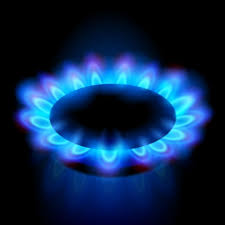Lecture slides     |     Basic equations     |      Text     |     Tools     |     Help

#### XXXHeat transferred at constant pressure## Study of the possible paths for an expansion led us to the conclusion that a constant pressure path defines a new state function. We will call that state function the enthalpy. Although we started by defining the constant volume state function, the internal energy, the constant pressure state function is even more useful. The reason is simple. We live in a world where the temperature is relatively constant. For routine use we want tabulated thermodynamic functions that we can use without applying corrections. Enthalpy is such a function.Heat capacityThe heat capacity is a measure of how much heat is required to raise the temperature of the sample by 1 degree. In a microscopic sense heat capacity depends on the availability of energy levels as temperature is increased. For example, translational energy levels are closely spaced. Their spacing is billions of times smaller than ambient thermal energy (RT). Therefore, a change in temperature results in a change in the population of translational energy levels. All gas phase molecules have a contribution to their heat capacity from translation. Rotational levels have a much greater energy spacing than translational levels, but they are still smaller than RT by a factor of 10 to 100 depending on the molecule (except for H2). The rotational levels too contribute to the heat capacity. However, typical vibrational energy levels have too great a spacing to contribute at ambient temperature. For example, a 1000 cm-1 mode has a quantum 5 times greater than RT (at 300 K). This means that the population of the first vibrational state is approximately exp(-5) or 0.0067. A change in temperature by 10 K to 310 K would change the population of 0.0079. This small change has a negligible effect on energy and therefore the vibrational population does not contribute to the heat capacity. In the heat capacity presentation we consider the definitions of heat capacity at constant volume and pressure and the use of heat capacity to calculate the enthalpy of reaction at temperatures other than 298 K, where the enthalpies are tabulated.Measuring heat capacity and enthalpyCalorimetry is the science of measuring changes in heat. The heat capacity presentation starts with a brief historical look at early calorimetry, which was mainly directed at measuring changes due to combustion. The so-called bomb calorimeter is used to measure enthalpies of combustion in a cosntant volume container. The container is filled with O2 and the sample is compressed into a pellet so that it will burn all at once. There is a small wire that permits electronic ignition. Then one measures the flow of heat from the combustion reaction into a water bath surrounding the metal bomb. More modern calorimetry uses two methods: Differential scanning calorimetry Isothermal titration calorimetry We will consider each of these in detail in the presentation on calorimetry.

problem solving### Practice as you go...### Calorimetry